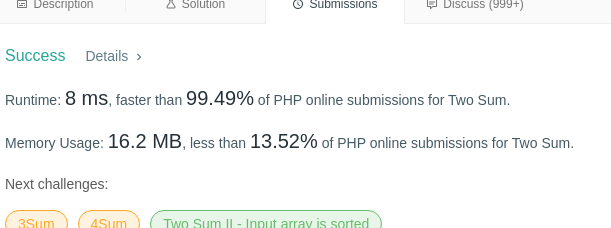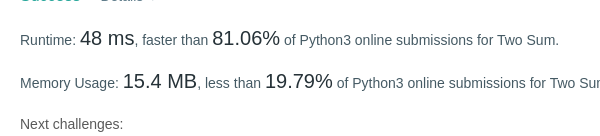#61

ahahahah

sorry

Здесь не могла быть ваша реклама
T7
53
#62

First, in the wrong steppe array_combine. Works, but not necessarily the first example gets c [1,2,3,3,3,3,3, 6].

Then it came. The point values as an index.

Option in the "forehead" slightly better memory, but slower

class Solution {
private \$ n, \$ t, \$ l;
public function twoSum (array \$ nums, int \$ target) {
\$ This-> n = \$ nums;
\$ This-> t = \$ target;
\$ This-> l = count (\$ nums);
\$ C = \$ this-> l-1;
for (\$ i = 0; \$ i <\$ c; \$ i ++) {
\$ R = \$ this-> calc (\$ this-> n [\$ i], \$ i + 1);
if (false == \$ r!) return [\$ i, \$ r];
}
return [];
}

private function calc (\$ val, \$ k) {
for (\$ i = \$ k; \$ i <\$ this-> l; \$ i ++) {
if (\$ this-> t == \$ val + \$ this-> n [\$ i]) return \$ i;
}
return false;
}
}
#63

a linear version https://pastebin.com/QJvnxc1A , but still far from the best solutions to php ..

png 1.png
#64

Gerga, very strange that the way the rough sorting overtaken 49% of all variants)), it is interesting that there are other ponapisyvali then) surely count in the cycle.

On pyhe I like this happened, but once he realized the algorithm becomes more not interested

Junior Web Developer
#65

I made their algorithm to pass tests, but still woefully bad. But this is still too much, though, and on heshtab, While I can not be cut in the algorithm (

Runtime: 5964 ms, faster than 8.52% of Python3 online submissions for Two Sum.
Memory Usage: 16 MB, less than 5.77% of Python3 online submissions for Two Sum.

---------- Posted 18.06.2020 at 17:47 ----------

Danforth:
http://imgur.com/28teQGyl.png

It's like this for 0 seconds? 🤪

T7
53
#66
Danforth:
On pyhe I like this happenedVery similar results

timo-71:
The point values as an index.
https://pastebin.com/NcuYkQbe
#67

timo-71, I have the same final decision. sotochku thought how to squeeze?

spoiler:

I thought at first that it is necessary preallotsirovat array (in Go and Rust I did), but this can not be done pyhe (SplFixedArray is still an array rather than Mapa or hash table).

T7
53
#68In Python with the same algorithm :(

---------- Posted 18.06.2020 at 19:06 ----------

Danforth:
sotochku thought how to squeeze?

That the idea is no more. :(

#69

Here is a C # https://dotnetfiddle.net/rX0UbZ

It seems to work correctly :)

Compile: 0.014s

Execute: 0.05s

Memory: 0b

CPU: 0.064s

⭐ Разработка Андроид-приложений (Xamarin C#). ⭐ Разработка ASP.NET (WebForms, MVC, WebAPI, Core). ⭐ Цой жив!